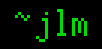## 26-Oct-2010

### Kinked pipes

Filed under: math — jlm @ 12:04

Our friend Keith Devlin poses an interesting question about expanding pipes: A pipe expands by one foot over its one-mile length; how high does it arch?

Keith assumes a single sharp kink in the center and uses Pythagoras to calculate that the pipe arches slightly over 50 feet! Yet if the pipe had its sharp kink near one end, it’d arch slightly under 1 foot. This suggests a calculus of variations problem: Of all the ways a pipe can bend, which way generates the highest arch? The dual problem would be: Of all the ways to arch to a given height (let’s say 50 feet), which way uses the least length of pipe?

Our pipe path will go from one pipe endpoint up to some point with height 50’, thence to the other pipe endpoint. If either of these paths weren’t a line segment, we could shorten the path by using a line segment. The angles the segments make with the horizontal line at 50’ will be equal, otherwise we could shorten the path by equalizing them. So the shortest path is a line up to the center 50’ up, kink, then a straight line to the ground at the other end.

Because this isoceles path is the shortest path to reach a given height, it’s also the highest-reaching path of its length. Keith’s assumption that the pipe sharply kinks in the center gives the highest arch of any possible path!

Minimizing the height isn’t so interesting: By making arbitrarialy fine accordion folds, we can keep the maximum height arbitrarialy small.

What if the pipe makes a parabolic arch? Depth below the arch height at distance x from the center is kx². For our case of a mile-long pipe, we have arch height k(2640)². The curve length is ∫02640 √(1+(2kx)²) dx. If you’re like me, you can’t integrate this without a reference, but it gives (2kx √(4k²x²+1)+sinh-1(2kx))/4k | x=2640. Numerically solving this for length 2640.5 gives k=6.385×10-6, which gives an arch height of 44.5 feet.

That was messier than I expected. I expect a catenary arch, which IIR my physics correctly is what a pipe should assume under gravity, would be much the same.

Why are the arches in the center so much higher than arches at the ends? Going back to the triangle case, if we increase our pipe length by an amount δ over a flat length x, we have height related by x²+h² = (x+δ)² from good old Pythagoras, which gives h = √(2xδ+δ²). δ will vary from near 1 at x=0 to near 0 at x=5280, δ ≈ 1-(x/5280), varies only slowly with x. So with the square root curve being steep at small values, at small x, x has a big impact on h! Toward the other end, δ’s decrease overpowers x’s increase, and as δ gets small h is pulled steeply down.

## 1 Comment

1. great post, thanks for sharing

Comment by Daniel — 17-Dec-2010 @ 12:22

Sorry, the comment form is closed at this time.